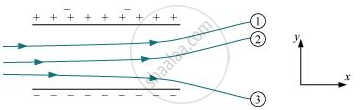# A Particle of Mass M And Charge (−q) Enters the Region Between the Two Charged Plates Initially Moving Along X-axis with Speed Vx - Physics

Numerical

A particle of mass m and charge (−q) enters the region between the two charged plates initially moving along x-axis with speed vx (like particle 1 in the fig.). The length of plate is L and an uniform electric field E is maintained between the plates. Show that the vertical deflection of the particle at the far edge of the plate is qEL2/(2m"v"_"x"^2).#### Solution

Charge on a particle of mass m = − q

Velocity of the particle = vx

Length of the plates = L

Magnitude of the uniform electric field between the plates = E

Mechanical force, F = Mass (m) × Acceleration (a)

"a" = "F"/"m"

However, electric force, F = qE

Therefore, acceleration, "a" = ("qE")/"m" ......(1)

Time taken by the particle to cross the field of length L is given by,

t = "lenght of the plate"/"Velocity of the particle" = "L"/"v"_"x" .......(2)

In the vertical direction, initial velocity, u = 0

According to the third equation of motion, vertical deflection s of the particle can be obtained as,

"s" = "ut" + 1/2"at"^2

"s" = 0 + 1/2("qE"/"m")("L"/"v"_"x")^2

"s" = ("qEL"^2)/(2"m""v"_"x"^2) ........(3)

Hence, the vertical deflection of the particle at the far edge of the plate is qEL2/(2m"v"_"x"^2).

This is similar to the motion of horizontal projectiles under gravity.

Concept: Coulomb’s Law - Force Between Two Point Charges
Is there an error in this question or solution?

#### APPEARS IN

NCERT Physics Part 1 and 2 Class 12
Chapter 1 Electric Charges and Fields
Exercise | Q 1.33 | Page 50
NCERT Class 12 Physics Textbook
Chapter 1 Electric Charge and Fields
Exercise | Q 33 | Page 50
Share